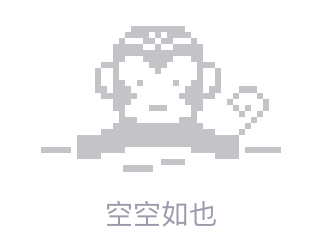# Dragon Ball

Problem Description
There are N "dragon balls" lined up into one row from left to right. Every dragon ball has two properties: the type and energy. We can cut these balls into some segments ( these segments should not be empty). You can not change the order of these balls. In each segment, if there exists a ball which is not the right most one and its type is the same with the right most one ,this segment will explode. The energy of a segment is the energy of the ball with largest energy in this segment. Now please minimize the total energy of all these segments without explosion.

Input
There will be T (T<=10) test cases. Each test case contains three lines. The first line comes an Integer N (1<=N<=100000), the number of dragon balls. The second line contains N integer numbers, indicating the type of the dragon balls. The types will be a number between 1 and 100000. The last line of each case also contains N integer numbers, representing the energy of each ball. The energy will be positive and not greater than 1000000.

Output
For each case, print a line contains a number representing the minimum energy value.

Sample Input
2
6
5 1 3 1 2 1
6 5 1 3 4 2
3
1 1 1
2 2 2

Sample Output
8
62017/10/29 09:54
• numbers
• each
• 点赞
• 收藏
• 回答
私信

#### 1个回复